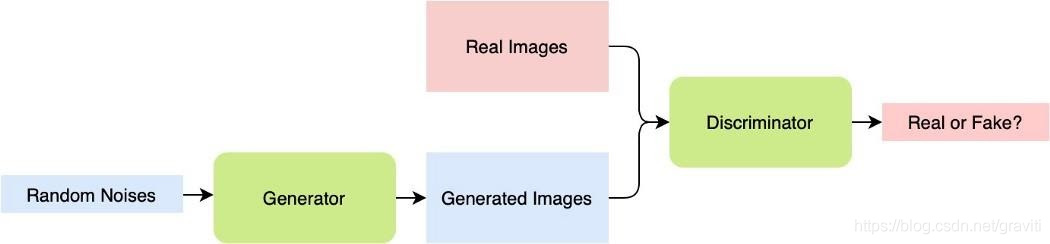Auto ByteScience AI# GAN的原理

Goodfellow等人则提出了一个有趣的解决办法：我们可以先训练好一个分类工具，来自动区分生成图像和真实图像。这样一来，我们就可以用这个分类工具来训练一个生成网络，直到它能够输出完全以假乱真的图像，连分类工具自己都没有办法评判真假。按照这一思路，我们便有了GAN：也就是一个生成器（generator）和一个判别器（discriminator）。生成器负责根据给定的数据集生成图像，判别器则负责区分图像是真是假。GAN的运作流程如上图所示。

# 计算环境

### 库

```"""
Import necessary libraries to create a generative adversarial network
The code is mainly developed using the PyTorch library
"""
import time
import torch
import torch.nn as nn
import torch.optim as optim
from torchvision import datasets
from torchvision.transforms import transforms
from model import discriminator, generator
import numpy as np
import matplotlib.pyplot as plt```

### 数据集

MNIST这样高质量的非结构化数据集都可以在格物钛公开数据集网站上找到。事实上，格物钛Open Datasets平台涵盖了很多优质的公开数据集，同时也可以实现数据集托管及一站式搜索的功能，这对AI开发者来说，是相当实用的社区平台。### 硬件需求

```"""
Determine if any GPUs are available
"""
device = torch.device('cuda' if torch.cuda.is_available() else 'cpu')```

# 实现

### 网络结构

```"""
Network Architectures
The following are the discriminator and generator architectures
"""

class discriminator(nn.Module):
def __init__(self):
super(discriminator, self).__init__()
self.fc1 = nn.Linear(784, 512)
self.fc2 = nn.Linear(512, 1)
self.activation = nn.LeakyReLU(0.1)

def forward(self, x):
x = x.view(-1, 784)
x = self.activation(self.fc1(x))
x = self.fc2(x)
return nn.Sigmoid()(x)

class generator(nn.Module):
def __init__(self):
super(generator, self).__init__()
self.fc1 = nn.Linear(128, 1024)
self.fc2 = nn.Linear(1024, 2048)
self.fc3 = nn.Linear(2048, 784)
self.activation = nn.ReLU()

def forward(self, x):
x = self.activation(self.fc1(x))
x = self.activation(self.fc2(x))
x = self.fc3(x)
x = x.view(-1, 1, 28, 28)
return nn.Tanh()(x)```

### 训练

```"""
Network training procedure
Every step both the loss for disciminator and generator is updated
Discriminator aims to classify reals and fakes
Generator aims to generate images as realistic as possible
"""
for epoch in range(epochs):
for idx, (imgs, _) in enumerate(train_loader):
idx += 1

# Training the discriminator
# Real inputs are actual images of the MNIST dataset
# Fake inputs are from the generator
# Real inputs should be classified as 1 and fake as 0
real_inputs = imgs.to(device)
real_outputs = D(real_inputs)
real_label = torch.ones(real_inputs.shape, 1).to(device)

noise = (torch.rand(real_inputs.shape, 128) - 0.5) / 0.5
noise = noise.to(device)
fake_inputs = G(noise)
fake_outputs = D(fake_inputs)
fake_label = torch.zeros(fake_inputs.shape, 1).to(device)

outputs = torch.cat((real_outputs, fake_outputs), 0)
targets = torch.cat((real_label, fake_label), 0)

D_loss = loss(outputs, targets)
D_loss.backward()
D_optimizer.step()

# Training the generator
# For generator, goal is to make the discriminator believe everything is 1
noise = (torch.rand(real_inputs.shape, 128)-0.5)/0.5
noise = noise.to(device)

fake_inputs = G(noise)
fake_outputs = D(fake_inputs)
fake_targets = torch.ones([fake_inputs.shape, 1]).to(device)
G_loss = loss(fake_outputs, fake_targets)
G_loss.backward()
G_optimizer.step()

if idx % 100 == 0 or idx == len(train_loader):
print('Epoch {} Iteration {}: discriminator_loss {:.3f} generator_loss {:.3f}'.format(epoch, idx, D_loss.item(), G_loss.item()))

if (epoch+1) % 10 == 0:
torch.save(G, 'Generator_epoch_{}.pth'.format(epoch))
print('Model saved.')```

# 不仅是有样学样

GAN和以往机器视觉专家提出的想法都不一样，而利用GAN进行的具体场景应用更是让许多人赞叹深度网络的无限潜力。下面我们来看一下两个最为出名的GAN延申应用。

### GauGAN# 结语